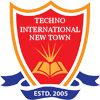#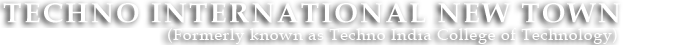+91 9674112076/2079
•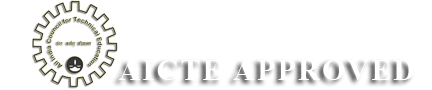•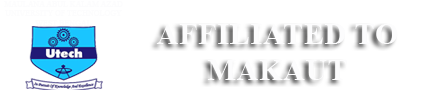•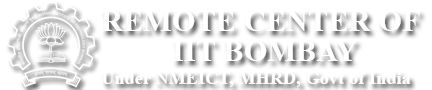•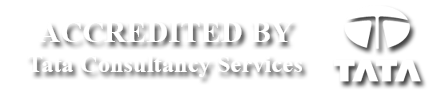# Analog and Digital Electronics Lab

ANALOG & DIGITAL ELECTRONIC CIRCUIT LABORATORY

Code :EC (EE) 391

List of Experiments:

1. Study of Ripple and Regulation characteristics of full wave rectifier with and without capacitor filter.
2. Study of Zener diode as voltage regulator.
3. Construction of two stage R-C coupled amplifier & study of its gain and Bandwidth.
4. Study of class A, C & Push pull amplifier.
5. Realization V-I & I-V converter using Operational Amplifier.
6. Study of timer circuit using NE 555 and configuration of Mono-stable and Astable Multivibrator.
7. Study of DAC & ADC
8. Realization of basic gates using Universal logic gates.
9. Realization of RS-JK & D filp-flop using logic gates.
10. Design of Combinational circuit for BCD to decimal conversion to drive 7-segment display using Multiplexer.
11. Realization of Synchronous Up/Down counter.
12. Construction of simple Decoder & Multiplexer circuits using logic gates.
14. Code conversion circuits- BCD to Excess-3 and vice-versa. (Innovative)
15. Four-bit parity generator and comparator circuits. (Innovative)

ANALOG & DIGITAL ELECTRONIC CIRCUIT LABORATORY

Code: CS-391

List of Experiments:

1. Design a Class A amplifier
2. Design a Phase-Shift Oscillator
3. Design of a Schmitt Trigger using 555 timer.
4. Design a Full Adder using basic gates and verify its output
5. Design a Full Subtractor circuit using basic gates and verify its output.
6. Construction of simple Decoder & Multiplexer circuits using logic gates.
7. Realization of RS / JK / D flip flops using logic gates.
8. Design of Shift Register using J-K / D Flip Flop.
9. Realization of Synchronous Up/Down counter.
10. Design of MOD- N Counter.
11. Study of DAC (R-2R ladder type).
12. Four-bit parity generator and comparator circuits. (Innovative)
13. Construction of adder circuit using Shift Register and full Adder. (Innovative)